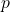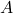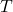# Turning Theory into Algorithms

Some of the common complaints I hear about (learning) theoretical work run along the lines of “those bounds are meaningless in practice,” “that result doesn’t apply to any algorithm someone would actually use,” and “you lost me as soon as martingales/Banach spaces/measure-theoretic niceties/… got involved.” I don’t have a good answer for the latter concern, but a very nice paper by Sasha Rakhlin, Ohad Shamir, and Karthik Sridharan at NIPS this year goes some ways toward address the first two criticisms. Their paper, “Relax and Randomize: From Value to Algorithms,” (extended version here) is concerned with transforming non-constructive online regret bounds into useful algorithms. The paper builds off previous statistical learning theory results for worst-case online learning by authors together with Ambuj Tewari. This preprint provides a summary of results for learning from sequential, dependent data. Their results mirror those for the i.i.d. setting, and  include necessary and sufficient conditions for uniform convergence of empirical processes with dependent data; notions of covering numbers, fat-shattering dimension, Rademacher complexity, and other measures of function class complexity in the sequential setting; and a variety of regret bounds based on these quantities.

The essential idea of Rahklin et al.’s approach to statistical learning theory for dependent sequences is to view learning as a 2-player game between the learner and Nature. This hearkens back to a classic way of doing worst-case analysis of online algorithms. In that scenario, the algorithm chooses some strategyand Nature (a.k.a. the Adversary) chooses some sequenceto give the algorithm. This results in some lossfor the algorithm. Forming a (possibly infinite) matrix, if the algorithm chooses a strategy according to(distribution over rows of) and Nature according to(distribution of columns), then we are interested in the value of the game,Here, the algorithm tries to minimize its loss while Nature tries to maximize the algorithm’s loss. The value of the game gives an upper bound for the loss (and hence performance) of the algorithm.

The situation is a little more complicated for online learning. Letbe the moves of the learner andbe the moves of Nature. For a finite number of rounds, the learner and Nature chooseandand then observe each other’s choices. These move pairs are used to define a loss function. The overall loss for the game is the regretThe second term relativizes the loss so that the algorithm is compared to how well you could have done if you had chosen the best possiblefrom the start. Unlike in the algorithms case, the value of the online learning “game” has interleaved min’s and max’s (well, inf’s and sup’s), since there arerounds in the game:whereis the set of all distributions over. In the learning scenario, the expectation plays the role asand Nature’s move can be deterministic while still giving the same value.

What Rahklin et al. do is consider relaxations, i.e. upper bounds, to(well, actually the bound a conditional form of). These relaxations are chosen to be more manageable to deal with analytically. At the-th stage of the game, instead of findingbased on the expression for, an approximateis calculated. Thisis the distribution overthat minimizes an expression that involves the maximum of the relaxation plus an expected loss term. Since the relaxation can be handled analytically, one now has an actual algorithm for calculating, which can be used to make a prediction. The authors derive sometimes improved versions of classic algorithms as well as some new ones. For example, they give  a parameter-free version of exponential weights which optimizes the learning rate in an online manner. Specifically, in round, for eachwhereis the optimal learning rate. The relaxation can then be used to bound the regret of the algorithm by. There are many other algorithms discussed, including mirror descent, follow the perturbed leader, and a matrix completion method. I highly recommend checking out the paper for the rest of the details and other examples.